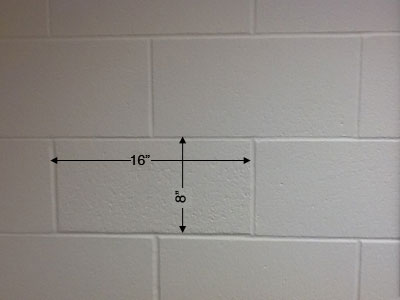SEARCH HOMEMath Central Quandaries & QueriesQuestion from david: hi how many concrete blocks would i need for an extension 50 ft long ,20 ft wide and 12ft heightHi David,

The concrete blocks that form one of the walls in my office measure 16 inches by 8 inches so each one covers an area of $16 \times 8 = 128$ square inches.The 50 foot long by 12 foot high wall you want to construct is $50 \times 12 = 600$ inches by $12 \times 12 = 144$ inches so its area is $600 \times 144$ square inches. Thus the number of blocks you need for this wall is

$\frac{600 \times 144}{128}.$

Perform the analogous calculation for the 20 foot walls and total the number of blocks you obtain. You will need to add a few extra as there will be some cutting and wastage at the corners.

PennyMath Central is supported by the University of Regina and The Pacific Institute for the Mathematical Sciences.